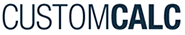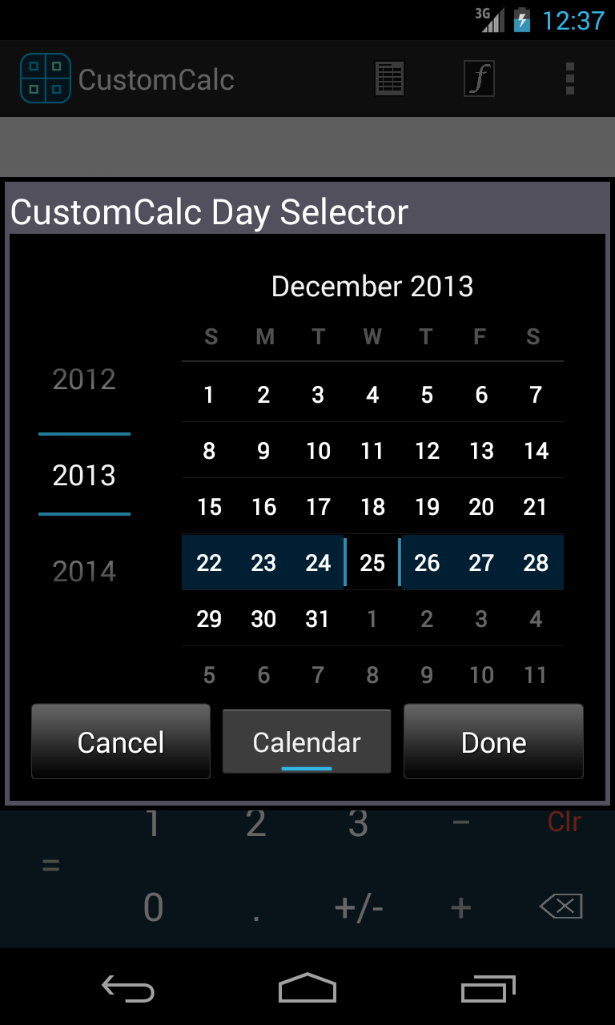﻿ CustomCalc - Android Date calculation••# Date and Time calculation

With CustomCalc you can work with dates and time in a very easy way, just as they are numbers in the display.

Working with days as a number, allows you to add days to a date, subtract days to a date, or subtracting two dates to find the days in between. To set a date you may enter a number in the format mm.ddyyy (can vary depending on your device settings) and press 'set Date' or by selecting a date though the 'Date' function'"

The same happens with time based functions, you may add to hms numbers, subtract them, or multiply and divide by a simple number. To enter a hms number just type it in the format h.mmss and press 'set hms'. Here you have also conversions from hours in decimals to hours-minutes and seconds and backwards.

From the keyboard,

• Date + x:
To add x days to a date introduced..
• Date - x:
To subtract x days to a date.
• Difference Between Days
• "DoW" Day of Week:
To return the Day Of Week for the date introduced
• "Date":
to select date:
• "Today":
Difference Between Days
• "to hr" Converts HMS to HR:
To convert a number in hms (hours, minutes & seconds) to decimal point hours (HR).
• "to hms" Convert HR to HMS:
To convert a number in decimal hours (HR), to HMS (hours, minutes & seconds).
• Add two numbers in HMS format.
• Subtract two numbers in HMS format.
• Multiply a HMS format number by x
• Divide a HMS format number by x